# Falloff Texture

The fall off map is a texture node typically used to control the blend of two materials depending on the viewing angle of the materials geometry. The Falloff map may be used to control the blending amount of a mixed node. The mixed node can either be a Mix Texture or a Mix MaterialThe representation of the surface or volume properties of an object.: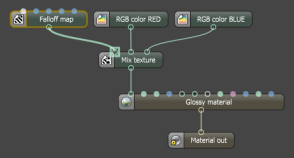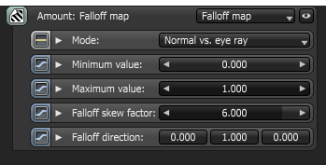The angle between the eye ray and the shading normal is mapped from [0°, 90°] to [0 ,1] For values larger than 1.0, the Falloff Node does a gamma correction using the skew factor as exponent. The skew factor is used to interpolate between the spectral shades resulting from the Minimum and Maximum values which are based on the first and second inputs of a mixed node.

Three modes of the Falloff Map

• Normal vs Eye Ray: This is the default mode where the falloff is calculated from the angle between the surface normal and the eye ray. This mode is more commonly used for reflections and the falloff color range affects faces directly in front of the view and gradually falls at angled faces towards the sides as it falls away from the straight on viewing angle therefore yhe Falloff Direction parameter does not apply.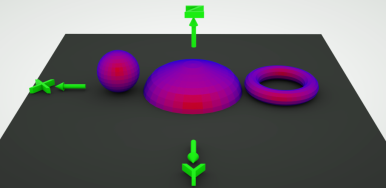(Skew factor = 1; Direction does not apply)

• Normal vs. Vector 90deg: The falloff is calculated from the angle between the surface normal and the specified direction vector maxing out at 90 degrees. This is similar to the default mode except that it maintains the effect of the color range according to the Falloff Direction x/y/z.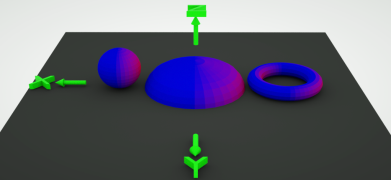(Skew factor = 1; Direction x=1)

• Normal vs. Vector 180deg: The falloff is calculated from the angle between the surface normal and the specified direction vector maxing out at 180 degrees. This provides a wider color range from the minimum to the maximum values and maintains the effect of the color range according to the Falloff Direction x/y/z.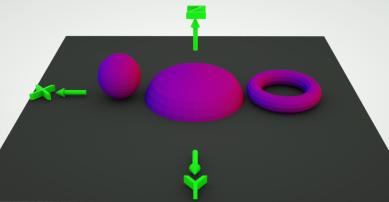(Skew factor = 1; Direction x=1)

Minimum Value
This is the value if the angle between the two directions is 0. This is the value/lightness (0 to 1) of the map at straight on viewing angles. When used as an input to a mix node, the Minimum Value is the normal spectral shade value (color range) between the first and second input.

Maximum Value
This is the value if the angle between the two directions is at the maximum. This is the value/lightness (0 to 1) of the map at grazing angles. When used as an input to a mix node, the Minimum Value is the grazing spectral shade value (color range) between the first and second.

Falloff Skew Factor
The relative amount of the Minimum and Maximum values that are at an angle to the straight on view. 0.1 will lead to almost complete coverage by the grazing value regardless of viewing angle, whereas 15 will lead to almost complete coverage by the normal value. This parameter is set at a default skew factor of 6.

While the index value on glossy and specular nodes corresponds to a real world IOR (Index of Refraction) value on dielectric materials, like plastic and glass (metals are not supported yet and OctaneRender also does not support Bezier curves), the falloff node works differently because of this falloff skew factor.

If set to 1 then the value is proportional to the angle between the normal and the camera ray, i.e. if it is viewed from 45° then the value is 0.5.
If larger than 1, then it applies a power curve to the angle.
If smaller than 1, then it inverts the skew factor, and mirrors the power curve.

_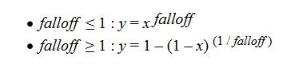_

Falloff Direction
The direction vector that is used by the Normal vs. Vector 90deg and Normal vs. Vector 180deg modes. For most materials, the fresnel effect (the default mode) is usually correct while falloff direction is used for exceptional cases which can be adjusted relative to the camera. Changing the rotation of the object will not change the orientation of the falloff direction.